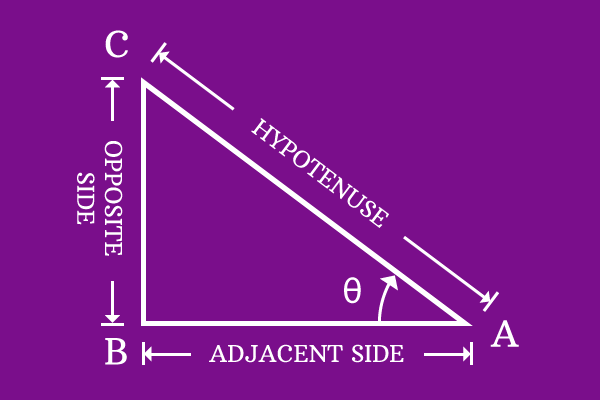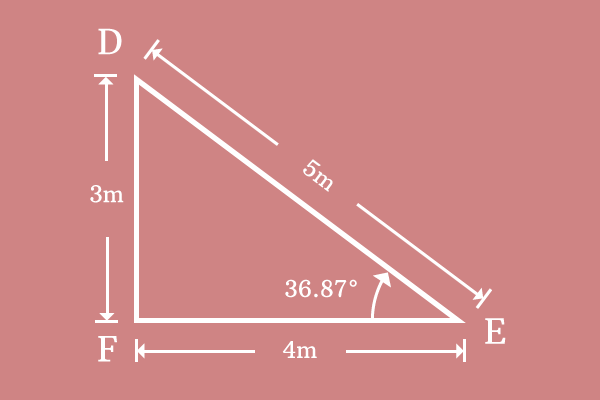# Tangent

## Definition

The relation of angle with ratio of length of opposite side to length of adjacent side in a right angled triangle is called tangent.Tangent reveals the mutual relation of angle of right angled triangle with ratio of length of opposite side to length of adjacent side. It is primarily defined from a ratio at an angle of right angled triangle in trigonometry. Hence, tangent is also called as trigonometric ratio.

Due to the functionality of representing the angle with ratio of length of opposite side to length of adjacent side, the trigonometric ratio tangent is also called as trigonometric function trigonometrically.

### Mathematical formThe name tangent was introduced in trigonometry to represent the relation of angle with ratio of length of opposite side to length of adjacent side. In fact, the ratio of length of opposite side to length of adjacent side is changed whenever the angle of right angled triangle is changed. The mutual dependency is expressed in mathematical language by writing tangent along with angle. In mathematics, tangent is expressed in its short form $tan$.

In the case of triangle $BAC$, the angle of this right angled triangle is theta $(\theta)$. Therefore, tangent along with angle is written as $tan \, \theta$ in mathematical language.

$$tan \, \theta = { Length \, of \, Opposite \, Side \over Length \, of \, Adjacent \, Side}$$

In $Δ BAC$, the length of opposite side $(\overline{BC})$ is $BC$ and length of adjacent side $(\overline{AB})$ is $AB$. Therefore, the mathematical expression can be expressed in terms of lengths of opposite side and adjacent side.

$$∴ \,\, tan \, \theta = { BC \over AB }$$

#### ExampleConsider right angled triangle $DEF$ and it is measured that

1. Length of the opposite side $(\overline{DF})$ is $DF = 3$ meters
2. Length of the adjacent side $(\overline{EF})$ is $EF = 4$ meters
3. Angle of the triangle $∠DEF$ is $36.87^°$

Substitute three values in the mathematical form expression of tangent.

$$⇒ tan \, 36.87^° = { DF \over EF }$$

$$⇒ tan \, 36.87^° = { 3 \over 4 }$$

$⇒ tan \, 36.87^° = 0.75$

The ratio of length of opposite side to length of adjacent side is $0.75$ at the angle of $36.87^°$. The value of ratio $0.75$ is called as value of tangent at the angle of $36.87^°$. Thus, it represents the relation of angle with ratio of length of opposite side to length of adjacent side in trigonometry.

Latest Math Topics
Latest Math Problems
Email subscription
Math Doubts is a best place to learn mathematics and from basics to advanced scientific level for students, teachers and researchers. Know more